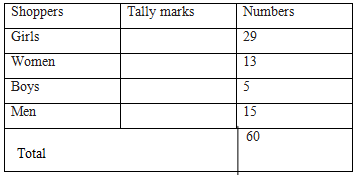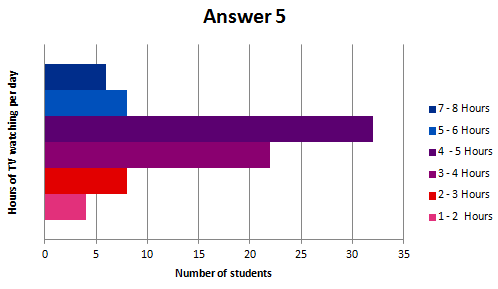Study Materials

# NCERT Solutions for Class 8th Mathematics

Page 1 of 3

## Chapter 5. Data Handling

### Exercise 5.1

1.   For which of these would you use a histogram to show the data? (a)   The number of letters for different areas in a postman’s bag.

(b)   The height of competitors in an athletics meet.

(c)   The number of cassettes produced by 5 companies.

(d)   The number of passengers boarding trains from 7:00 a.m. to 7:00 p.m. at a station.

Give reasons for each.

Ans.

In the case of the data given in alternative (b) and (d), we will use histogram as we can divide

The given data in class intervals. In case of alternative (a) and (c), we do not know about the numbers of letter of different areas and the number of cassettes produces by given companies. We don’t have any approximate idea about it. Therefore, we cannot define class intervals for this data and thus we will note use a histogram.

2.   The shoppers who come to a departmental store are marked as: man (M), woman (W), boy (B) or girl (G). The following list gives the shoppers who came during the first hour in the morning:

W W W G B W W M G G M M W

W W W G B M W B G G M W W M M W W W M W B W G M W W W G W M M W W M W G W M G W M M B G G W

Ans:

By observing the data above, we can make a frequency distribution table as follows.3.   The weekly wages (in `) of 30 workers in a factory are.

830, 835, 890, 810, 835, 836, 869, 845, 898, 890, 820, 860, 832, 833, 855, 845,

804, 808, 812, 840, 885, 835, 835, 836, 878, 840, 868, 890, 806, 840

Using tally marks make a frequency table with intervals as 800–810, 810–820 and so on.

Ans:1. 830-840 is the group which has maximum number of workers.
2. The worker who earn more than Rs 850 are the number of workers who fall in the group of 800-810 or 810-820 or 820-830 or 830-840 or 840-850 Hence the total number of workers earning less than 850 will be the sum of the numbers of all these workers i.e. 3 + 2 + 1 + 9 + 5 = 20

5.   The number of hours for which students of a particular class watched television during holidays is shown through the given graph.

Answer the following.

1. for how many hours did the maximum number of students watch TV?
2.   How many students watched TV for less than 4 hours?
3. How many students spend more than 5 hours in watching TV?

# Answer:

1. From the graph, it can be observe that the maximum number of students watched TV for 4 – 5 hours.
2. The students who watched TV for less than 4 hours are students who watched TV for  1-2 hours or 2-3 hours or 3-4 hours.

Hence the total number of students = 4 + 8 + 22 = 34

1. The students who watched TV for more than 5 hours are students who watched TV for 5-6 hours or 6-7 hours.

Hence, total number of students = 8 + 6 =  14Page 1 of 3

Chapter Contents: